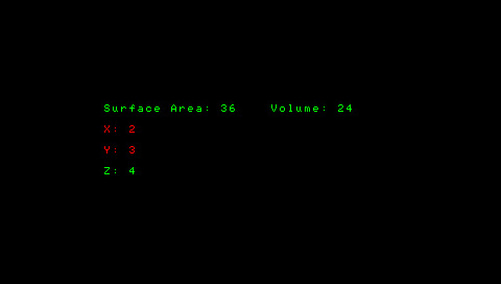# AutoGrapher PSP

### From GameBrew - a wiki dedicated to Video Game Homebrew.

General AutoGrapher10\$man Math 1.5 Update (27 Apr 2011) Mixed Download Website

AutoGrapher is an application to assist calculating Algebra 1 and a small portion of Geometry. It is made in Lua by 10\$man.

This was submitted to the PSP Genesis Competition 2011.

## Features

• Factor a list of Polynomials and solve them for 0 (10 polynomials at a time to be exact, value has to be set on your computer).
• Factor a single polynomial that you input on your psp.
• FOIL (or Distribute) like un-factoring.
• Find the Volume and Surface Area of: Cube, Rectangular Prisms, Cylinders.
• Graph.
• Beneksturun Theorom.

## Installation

Developer noted that you must have your PSP set to 1.50 if you are using a PSP1000.

## User guide

• Goto Graph.
• Goto Beneksturun.
• Goto Polynomial.
• Quick Solve Factoring Polynomials.
• Solve Already Input Factoring Polynomials.
• FOIL Polynomials.
• Go Back.
• Goto Formulas.
• Cube.
• Rectangular Prism.
• Cylinder.
• Back.
• Credits.
• Settings.

### Finding Volume and Surface Area

Goto Formulas> Cube, enter the value of the height and width and it will give you the answer automatically.

Same steps apply for Rectangular Prism and Cylinder.

### How to use the graph

From your computer navigate to your PSP and locate the folder /PSP/GAME/AutoGrapher/System/.

```---GRAPHS GO HERE---
y1=-x^3
y2=x+1
y3=x^5

```

Then start AutoGrapher> Goto Graph, and you will be able to move the tracer.

### Solving 10 Polynomials at a time

From your computer navigate to your PSP and locate the folder /PSP/GAME/AutoGrapher/System/.

```num1=16
num2=28

num1=20
num2=36

num1=2
num2=-8

num1=-3
num2=-28

num1=-19
num2=48

```

```X^2+16X+28

```

Set each of the num1 num2 pairs like this:

```set num1=16
and num2=28

```

Then run the application to get the answer, if it stays 0,0 it is prime.

### How to use Polynomial Quick Solve

Goto Quick Solve Factoring Polynomials on your PSP and enter in the equation. For example,

```2X^2+7X+5

```

Set:

```value1=2
value2=7
value3=5

```

Use the Left and Right button to change the number; Down and Up button to change the values.

Confirm then wait for it to tell you Done or Prime.

## Controls

D-Pad Up/Down - Choose between option, Change value (Polynomial)

D-Pad Left/Right - Move tracer (Graph), Change number (Polynomial), Change vlaue (Formulas)

Cross - Perform the marked action, Confirm

## Compatibility

Developer noted it should work on PSP2000.

Tested on:

PSP Brite TN-D
PSPGo
PSP Slim 5.00m33-6
PSP Phat 5.00m33-6

## Changelog

v1.5

• Polynomial solver should work fine now.
• UMD quick start.
• Other small fixes.

## Credits

Thank you to w00tguy123 for helping with the Graph.